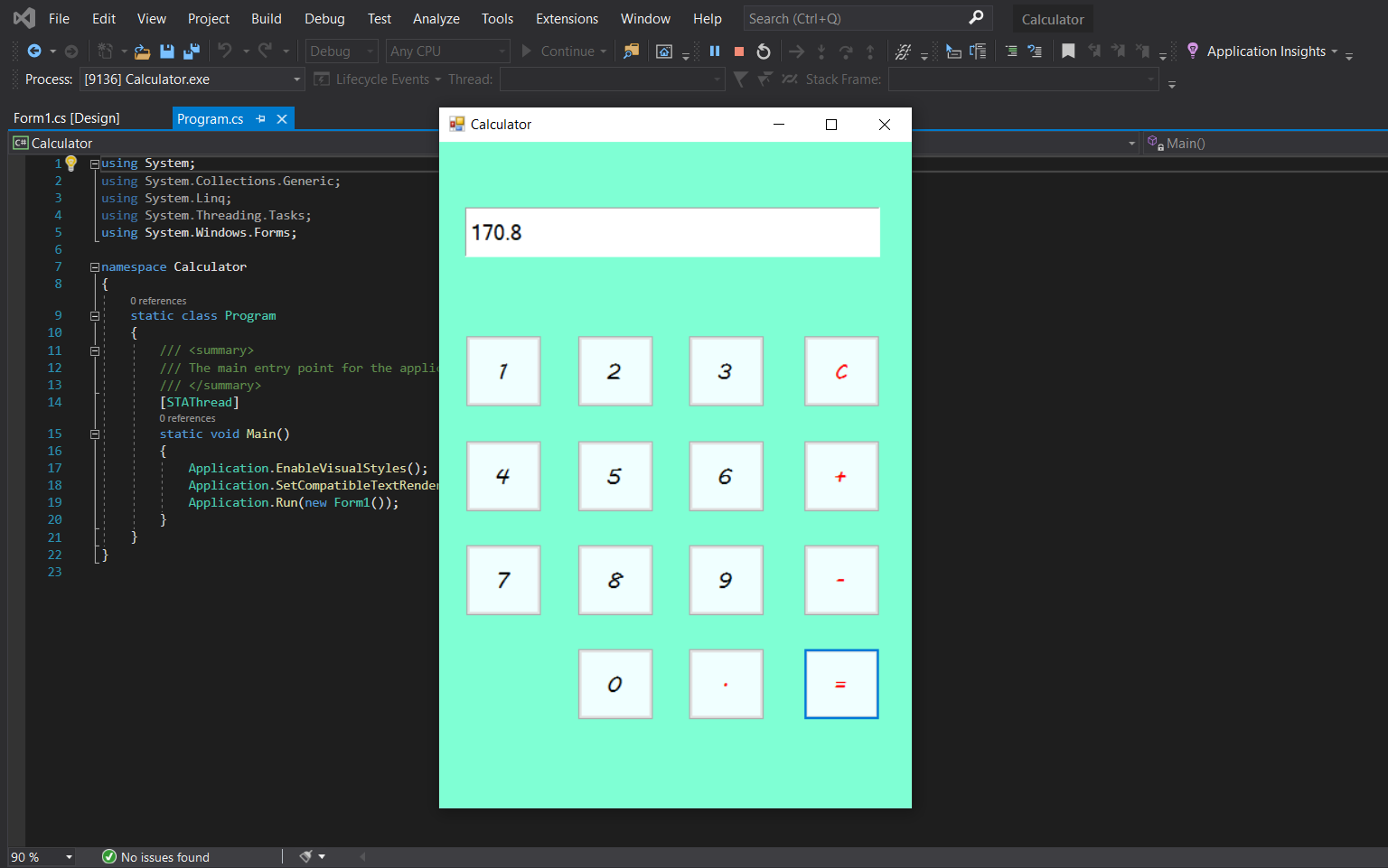# Simple Calculator In C# With Source Code

Project: Simple Calculator in C# with source code

Simple Calculator in C# is based on the concept of normal mathematical operations on the calculator. The project contains the numbers, operators, and signs like in a normal calculator. So, the user cannot enter the number themselves, they can just click on the numbers they want in the calculations. Hence, the user can use a simple calculator from this application.